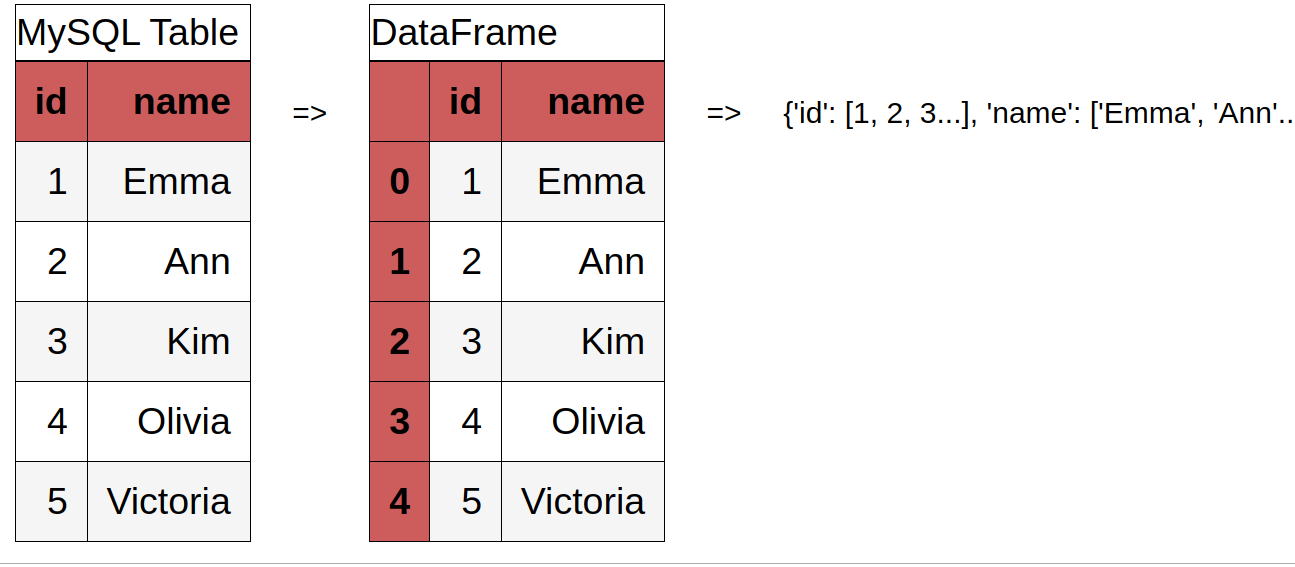In this short tutorial we will convert MySQL Table into Python Dictionary and Pandas DataFrame. If you need the reverse operation - convert Python dictionary to SQL insert then you can check:

In this tutorial we will use several Python libraries like:

• PyMySQL + SQLAlchemy - the shortest and easiest way to convert MySQL table to Python dict
• mysql.connector
• pyodbc in order to connect to MySQL database, read table and convert it to DataFrame or Python dict.## 1. Python convert MySQL table to Pandas DataFrame (to Python Dictionary) with SQLAlchemy

### 1.1. Install libraries SQLAlchemy + PyMySQL

Let's install dependencies required for Python in order to connect to MySQL database - in this case - PyMySQL + SQLAlchemy:

``````pip install PyMySQL
pip install SQLAlchemy
``````

### 1.2 Connect to MySQL database

``````from sqlalchemy import create_engine
import pymysql

db_connection_str = 'mysql+pymysql://root:pass@localhost:3306/test'
db_connection = create_engine(db_connection_str)
``````

Now we are going to connect to `localhost:3306`, database is `test` and the `user`/`password` are: root/pass.

### 1.3 Convert SQL table to DataFrame with SQLAlchemy

``````df = pd.read_sql('SELECT * FROM girls', con=db_connection)
``````

### 1.4. Convert SQL DataFrame to dictionary

The final step is to convert a DataFrame to Python dictionary by:

``````dict1 = df.to_dict('records')
dict2 = df.to_dict('list')
``````

where:

• ‘records’ : list like [{column -> value}, … , {column -> value}]
• ‘list’ : dict like {column -> [values]}
• ‘dict’ (default) : dict like {column -> {index -> value}}
• ‘records’ : list like [{column -> value}, … , {column -> value}]

### 1.5. Full Code: MySQL table to Python dict

``````from sqlalchemy import create_engine
import pymysql

db_connection_str = 'mysql+pymysql://root:kostov@localhost:3306/test'
db_connection = create_engine(db_connection_str)

df = pd.read_sql('SELECT * FROM girls', con=db_connection)
df.to_dict('list')
``````

result:

`{'id': [1, 2, 3, 4, 5], 'name': ['Emma', 'Ann', 'Kim', 'Olivia', 'Victoria']}`

### 1.6. Control dictionary output

• `df.to_dict()`
``````[{'id': 1, 'name': 'Emma'},
{'id': 2, 'name': 'Ann'},
{'id': 3, 'name': 'Kim'},
{'id': 4, 'name': 'Olivia'},
{'id': 5, 'name': 'Victoria'}]
``````
• `df.to_dict('list')`
``````{'id': [1, 2, 3, 4, 5], 'name': ['Emma', 'Ann', 'Kim', 'Olivia', 'Victoria']}
``````
• `df.to_dict('records')`
``````[{'id': 1, 'name': 'Emma'},
{'id': 2, 'name': 'Ann'},
{'id': 3, 'name': 'Kim'},
{'id': 4, 'name': 'Olivia'},
{'id': 5, 'name': 'Victoria'}]
``````
• `df.to_dict('index')`
``````{0: {'id': 1, 'name': 'Emma'},
1: {'id': 2, 'name': 'Ann'},
2: {'id': 3, 'name': 'Kim'},
3: {'id': 4, 'name': 'Olivia'},
4: {'id': 5, 'name': 'Victoria'}}
``````

## 2. Convert MySQL Table to Pandas DataFrame with mysql.connector

### 2.1. Install mysql-connector

To start lets install the latest version of `mysql-connector` - more info - MySQL driver written in Python by:

``````pip install mysql-connector
``````

### 2.2. Connect to MySQL database with mysql.connector

The connection to MySQL database requires several properties to be set:

• `host` - the host IP, usually localhost or 127.0.0.1
• `user` - the user which will be connected to the MySQL
• `password` - the password in plain text
• `database` - which database will be used

The code below shows the connection to MySQL database - `test` with user `root` without password:

``````import pandas as pd
import mysql.connector

db = mysql.connector.connect(
host="localhost",
user="root",
database="test"
)
``````

### 2.3. Read MySQL table by SQL query into DataFrame

Now we can query data from a table and load this data into DataFrame. All we need to do is to create a cursor and define SQL query and execute it by:

``````cur = db.cursor()
sql_query = "SELECT * FROM girls"
cur.execute(sql_query)
``````

Once data is fetched it can be loaded into DataFrame or consumed:

``````df_sql_data = pd.DataFrame(cur.fetchall())
``````

Note: If you have problems with encoding or data is shown as `bytearray(b'Kim'),` you can try to use instead of package `mysql-connector` with `mysql-connector-python`:

``````pip install mysql-connector-python
``````

### 2.5. Convert MySQL table to Python dictionary full code

``````import pandas as pd
import mysql.connector

db = mysql.connector.connect(
host="localhost",
user="root",
database="test"
)

cur = db.cursor()

cur.execute("SELECT * FROM girls")
df_sql_data = pd.DataFrame(cur.fetchall())

db.close()

df_sql_data.to_dict()
``````

## 3. Connect to MySQL DataBase with PyODBC

### 3.1. Install PyODBC on Ubuntu

The installation guide for PyODBC is available here: pyodbc - Installing on Linux.

In short in Ubuntu you need to install dependencies `unixodbc-dev` by writing in terminal:

``````sudo apt install unixodbc-dev
``````

If this is not install you will get error during installation of the PyODBC:

`fatal error: sql.h: No such file or directory`

Next step is to install the Python library in your virtual environment or Python by:

``````pip install pyodbc
``````

### 3.2. Install MySQL ODBC driver

Depending on your system you might have drivers installed or not. You can check do you have drivers by:

``````pyodbc.drivers()
``````

If you get an empty list then you need to install Driver. For MySQL and Ubuntu 18 you can find the instructions here:

Install mysql odbc drivers in ubuntu 18.04: Install mysql odbc drivers in ubuntu 18.04

### 3.3. Convert MySQL table to Pandas DataFrame with PyODBC

The final step is to** connect to MySQL database by using code** like the one below:

``````import pandas as pd
import pyodbc

cnn = pyodbc.connect("DRIVER={MySQL};SERVER=localhost;PORT=3306;DATABASE=test;UID=root;PWD=test;CHARSET=utf8;")

sql = 'select * from table'
There are two main points here. The first one is building the connection string - `cnn` where: